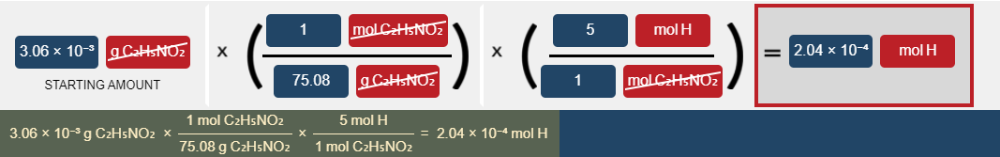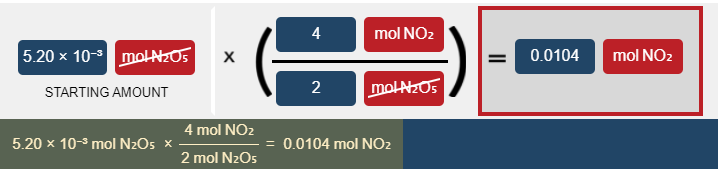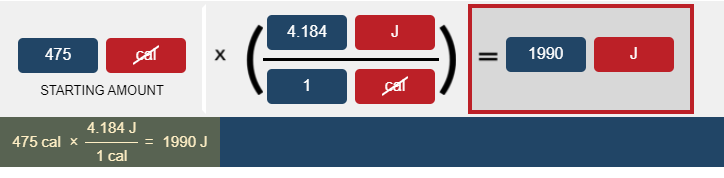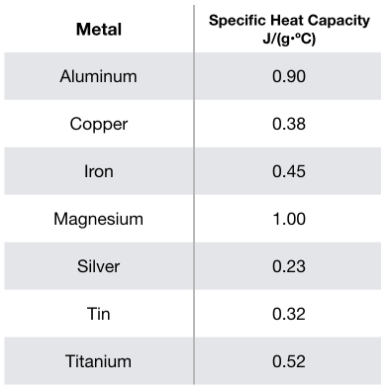# Quiz 3 - Flashcards

Set Details Share
created 3 years ago by SkyRose
5,276 views
Subjects:
chemistry
Page to share:
Embed this setcancel
COPY
code changes based on your size selection
Size:
X

1

Balance the following chemical equation (if necessary) for the combustion reaction of propane:

C₃H₈(g) + O₂(g) → CO₂(g) + H₂O(g)

C₃H₈(g) + 5O₂(g) → 3CO₂(g) + 4H₂O(g)

2

Balance the following chemical equation (if necessary):

C₃H₆(g)+O₂(g)→CO₂(g)+H₂O(g)

2 C₃H₆(g) + 9 O₂(g) → 6 CO₂(g) + 6 H₂O(g)

3

Balance the following chemical equation (if necessary):

H₂SO₄(aq)+Pb(OH)₄(s)→Pb(SO₄)₂(s)+H₂O(l)

2H₂SO₄(aq) + Pb(OH)₄(s) → Pb(SO₄)₂(s) + 4H₂O(l)

4

Balance the following chemical equation (if necessary):

Si₂H₃(s)+O₂(g)→SiO₂(g)+H₂O(g)

4 Si₂H₃(s) + 11 O₂(g) → 8 SiO₂(g) + 6 H₂O(g)

5

Balance the following chemical equation (if necessary):

KOH(aq)+H₂S(aq)→H₂O(l)+K₂S(aq)

2KOH(aq) + H₂S(aq) → 2H₂O(l) + K₂S(aq)

6

Balance the following chemical equation (if necessary):

Zn(s)+AgNO₃(aq)→Ag(s)+Zn(NO₃)₂(aq)

Zn(s) + 2AgNO₃(aq) → 2Ag(s) + Zn(NO₃)₂(aq)

7

Balance the following chemical equation (if necessary):

KClO₃(s)→KCl(s)+O₂(g)

2KClO₃(s)→ 2KCl(s) + 3O₂(g)

8

Write a balanced chemical equation based on the following description: aqueous iridium(III) bromide reacts with aqueous silver acetate to form solid silver bromide and aqueous iridium(III) acetate

IrBr₃(aq) + 3 AgC₂H₃O₂(aq) → 3 AgBr(s) + Ir(C₂H₃O₂)₃(aq)

9

Write a balanced chemical equation based on the following description: gaseous nitrogen and hydrogen react to form gaseous ammonia

N₂(g) + 3 H₂(g) → 2 NH₃(g)

10

Write a balanced chemical equation based on the following description: solid potassium oxide reacts with liquid water to produce aqueous potassium hydroxide

K₂O(s) + H₂O(l) → 2 KOH(aq)

11

Write a balanced chemical equation based on the following description: the reaction of solid carbon with oxygen gas to form carbon dioxide gas.

C(s) + O₂(g) → CO₂(g)

12

What is the coefficient in front of the NO when the equation below is balanced? NH₃(g) + O₂ (g) → NO(g) + H₂O (g)

4

13

How many oxygen atoms are located on the reactant side of the following chemical equation?

2 Al(s) + Fe₂O₃(s) → Al₂O₃(s) + 2 Fe(l)

A) 1

B) 2

C) 3

D) 4

E) 5

C) 3

14

Which of the following represents the generic form of a single-displacement reaction?

A) A + BX → AX + B

B) AB → A + B

C) AX + BY → AY + BX

D) A + B → AB

A) A + BX → AX + B

15

Which of the following represents the generic form of a synthesis reaction?

A) A + BX → AX + B

B) A + B → AB

C) AB → A + B

D) AX + BY → AY + BX

B) A + B → AB

16

Which of the following reactions is a double displacement reaction?

A) HCl (g) → H₂ (g) + Cl₂ (g)

B) HCl (aq) + NaOH (aq) → H₂O (l) + NaCl (aq)

C) Mg (s) + 2HCl (aq) → MgCl₂ (aq) + H₂ (g)

D) HCl (g) + C₅H₁₀ (g) →C₅H₁₁Cl (g)

B) HCl (aq) + NaOH (aq) → H₂O (l) + NaCl (aq)

17

What type of reaction is represented by the following equation: C₄H₈(g) + 6O₂(g) → 4CO₂(g) + 4H₂O(g)

A) acid-base

B) decomposition

C) precipitation

D) redox (oxidation-reduction)

D) redox (oxidation-reduction)

18

What type of reaction is represented by the following equation: SiCl₄(l) + 2Mg(s) → 2MgCl₂(s) + Si(s)

A) combination

B) decomposition

C) double displacement

D) single displacement

D) single displacement

19

In the following reaction, which element in what species is oxidized? C₂H₄ (g) + 3 O₂ (g) → 2 CO₂ (g) + 2 H₂O (g)

A) C in C₂H₄

B) H in C₂H₄

C) O in O₂

D) This is not an oxidation/reduction type of reaction.+

A) C in C₂H₄

20

In the following reaction, which element in what species is reduced? Zn (s) + 2 HCl (aq) → ZnCl₂ (aq) + H₂ (g)

A) Zn

B) H in HCl

C) Cl in HCl

D) This is not an oxidation/reduction type of reaction.

B) H in HCl

21

Determine the mass, in grams, of 0.400 moles of Pb (1 mol of Pb has a mass of 207.2 g).

82.9 g

22

Determine the number of atoms in 51.0 grams of sodium, Na. (The mass of one mole of sodium is 22.99 g.)

1.34 × 10²⁴ atoms

23

How many moles of calcium atoms do you have if you have 3.00 × 10²¹ atoms of calcium. (The mass of one mole of calcium is 40.08 g.)

0.00498 mol

24

Determine the molar mass of Ag₃N.

337.62 g/mol

25

How many moles of hydrogen are in 3.06 × 10⁻³g of glycine, C₂H₅NO₂?2.04 × 10⁻⁴ mol H.

26

How many hydrogen atoms are in 0.1488g of phosphoric acid, H₃PO₄?

2.743 × 10²¹ atoms H.

27

How many atoms are in 1.2 mol of carbon?

7.2 × 10²³ atoms

28

Which of the following contains the most moles of atoms?

A) 1 g of helium

B) 1 g of carbon

C) 1 g of uranium

D) All contain equal number of atoms

E) Not enough information+

A) 1 g of helium

29

Determine the number of atoms of O in 7.23 moles of Ca(NO₃)₂.

2.61 × 10²⁵ atoms

30

What is the mass grams that are in 1 molecule of H₂?

3.34 × 10⁻²⁴ g

31

According to the balanced reaction below, calculate the moles of NO₂ that form when 5.20 × 10⁻³mol of N₂O₅ completely reacts: 2N₂O₅(g)→4NO₂(g)+O₂(g)0.0104 mol NO₂.

32

Determine the number of molecules of Cr₂O₃thatformwhen1.34 × 10³g of oxygen completely reacts according to the following equation: 4Cr(s)+3O₂(g)→2Cr₂O₃(s)

1.68 × 10²⁵ molecules Cr₂O₃.

33

How many moles of MnO₃ are produced when 4.30kg of oxygen gas completely reacts according to the balanced chemical reaction: 2Mn(s)+3O₂(g)→2MnO₃(s)

89.6 mol MnO₃.

34

How many moles of nitrogen gas would be produced if 3.40 moles of copper(II) oxide were reacted with excess ammonia in the following chemical reaction? 2 NH₃(g) + 3 CuO (s) → 3 Cu(s) + N₂(g) + 3 H₂O(g)

1.13 mol

35

What is the mass in grams of NO that will be produced from 30.0 g of NO₂ reacted with excess water in the following chemical reaction? 3 NO₂(g) + H₂O(l) → 2 HNO₃(g) + NO(g)

6.52 g

36

If you have 5 mol H₂ and 2 mol N₂, what is the limiting reagent in the reaction below? 3 H₂(g) + N₂ (g) → 2 NH₃ (g)

A) H₂

B) N₂

C) NH₃

D) Both reactants are limiting.

A) H₂

37

If 50.0 g of H₂ and 100.0 g of O₂ react, how many moles of H₂O can be produced in the reaction below? 2 H₂(g) + O₂(g) → 2 H₂O(g)

6.250 mol

38

If 34.7 g of AgNO₃ react with 28.6 g of H₂SO₄ according to this UNBALANCED equation below, what is the mass in grams of Ag₂SO₄ that could be formed? AgNO₃(aq) + H₂SO₄ (aq) → Ag₂SO₄ (s) + HNO₃ (aq)

31.8 g

39

If 10.0 moles of O₂ are reacted with excess NO in the reaction below, and only 4.2 mol of NO₂ were collected, then what is the percent yield for the reaction? 2 NO (g) + O₂ (g) → 2 NO₂ (g)

21 %

40

In the reaction below, 7.0 mol of NO and 5.0 mol of O₂ are reacted together. The reaction generates 3.0 mol of NO₂. What is the percent yield for the reaction? 2 NO (g) + O₂ (g) → 2 NO₂ (g)

43 %

41

Convert 475 cal to joules1990 J

42

How many Joules are there in 2001 calories? (1 cal = 4.184 J)

8.372 × 10³ J

43

How many calories are in 1001 kJ ? (1 cal = 4.184 J)

2.392 × 10⁵ cal

44

When molecules absorb heat, there is an increase in

A) the kinetic energy of the molecules.

B) The potential energy of the molecules.

C) The mass of the molecules.

D) The bond energy of the molecules.

A) the kinetic energy of the molecules.

45

Which of the following represents an example of kinetic energy?

A) a ball at the top of a hill.

B) A ball rolling down a hill.

C) A ball at the bottom of a hill.

B) A ball rolling down a hill.

46

Which one of the following processes is exothermic?

A) Boiling a liquid.

B) Condensation of a gas into a liquid.

C) Melting a solid.

D) Converting solid into gas.

B) Condensation of a gas into a liquid.

47

In which one of the following processes is the system endothermic?

A) A process in which the air around the reaction becomes warmer.

B) A process taking place in solution where the solution temperature decreases.

C) The combustion of natural gas.

D) The formation of frost on a window.

B) A process taking place in solution where the solution temperature decreases.

48

Which one of the equations below is an endothermic reaction?

A) SrO (s) + CO₂ (g) → SrCO₃ (s)∆H° = -234 kJ/mol

B) H₂ (g) + F₂ (g) → 2 HF (g) ∆H° = -79.2 kJ/mol

C) H₂ (g) + C (s) + N₂ (g) → 2 HCN (g) ∆H° = 270.3 kJ/mol

D) 2 K (s) + 2 H₂O (l) → 2 KOH (aq) + H₂ (g) ∆H° = -393.1 kJ/mol

C) H₂ (g) + C (s) + N₂ (g) → 2 HCN (g) ∆H° = 270.3 kJ/mol

49

If a reaction favors products over reactants more as heat is added, that reaction is:

A) exothermic

B) endothermic

C) isobaric

D) isochoric

B) endothermic

50

How much heat (in kJ) will be absorbed by a 50.3 g piece of aluminum (specific heat = 0.930 J/g･°C) as it changes temperature from 23.0°C to 67.0°C?

2.06 kJ

51

An unknown solid is added to water in a calorimeter. The temperature of the water increases. What does this say about the reaction?

A) The reaction is exothermic.

B) The reaction is endothermic.

C) The reaction has absorbed heat from the solution.

D) The reaction has released heat to the solution.

E) Both A and D.

E) Both A and D.

52hat is the identity of a 100. g sample of metal that, upon absorbing 4680 J of heat, increases in temperature by 52.0°C?

A) Mg

B) Al

C) Ti

D) Fe

B) Al

53

Which one of the following phase changes would be exothermic?

A) freezing

B) boiling

C) sublimation

D) melting

A) freezing